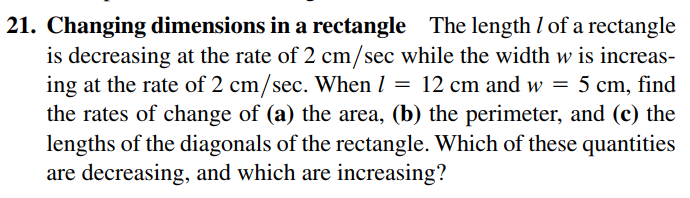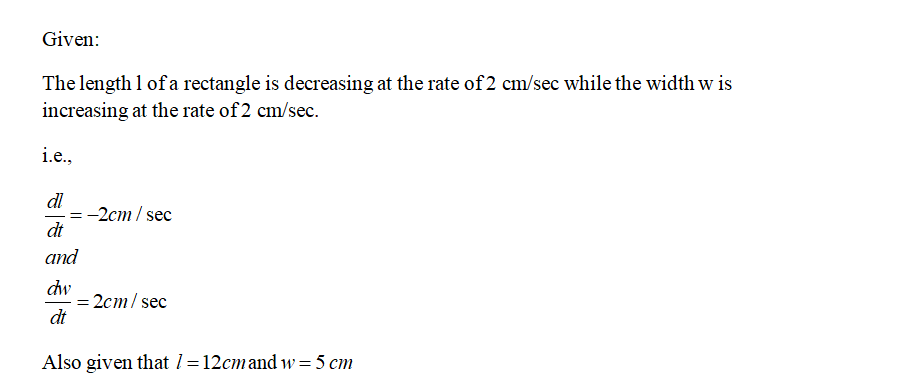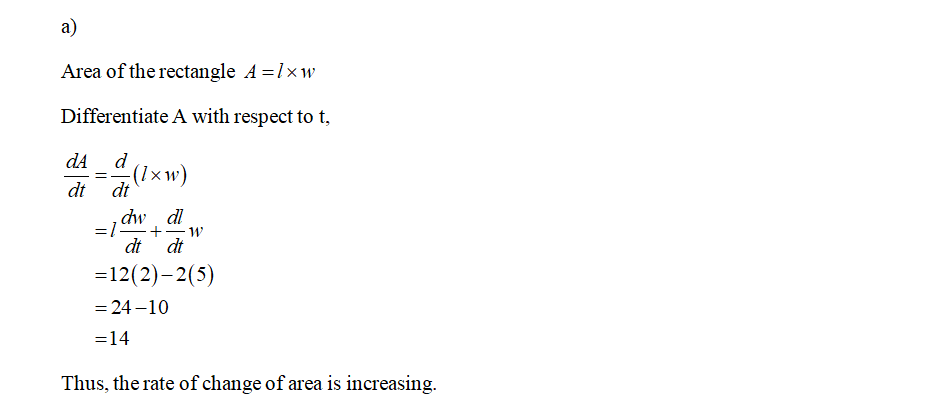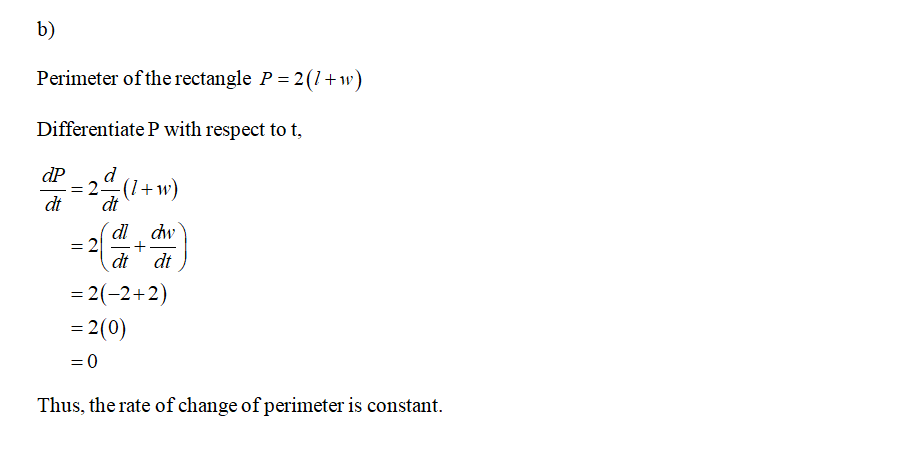# 21. Changing dimensions in a rectangle The length I of a rectangleis decreasing at the rate of 2 cm/sec while the width w is increas-ing at the rate of 2 cm/sec. When I = 12 cm and w = 5 cm, findthe rates of change of (a) the area, (b) the perimeter, and (c) thelengths of the diagonals of the rectangle. Which of these quantitiesare decreasing, and which are increasing?

Question
2 views

Can i get help with this problem step by stephelp_outlineImage Transcriptionclose21. Changing dimensions in a rectangle The length I of a rectangle is decreasing at the rate of 2 cm/sec while the width w is increas- ing at the rate of 2 cm/sec. When I = 12 cm and w = 5 cm, find the rates of change of (a) the area, (b) the perimeter, and (c) the lengths of the diagonals of the rectangle. Which of these quantities are decreasing, and which are increasing? fullscreen
check_circle

Step 1Step 2Step 3...

### Want to see the full answer?

See Solution

#### Want to see this answer and more?

Solutions are written by subject experts who are available 24/7. Questions are typically answered within 1 hour.*

See Solution
*Response times may vary by subject and question.
Tagged in